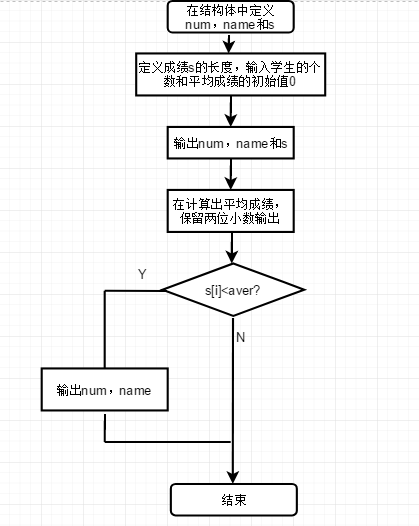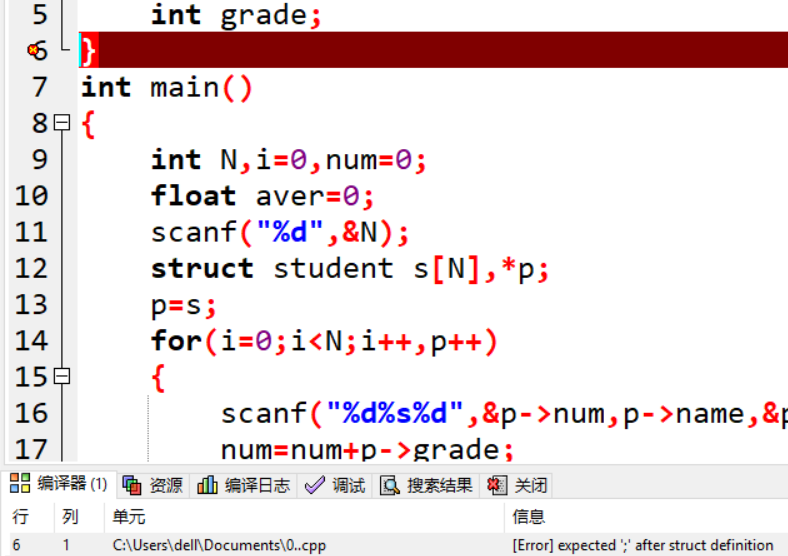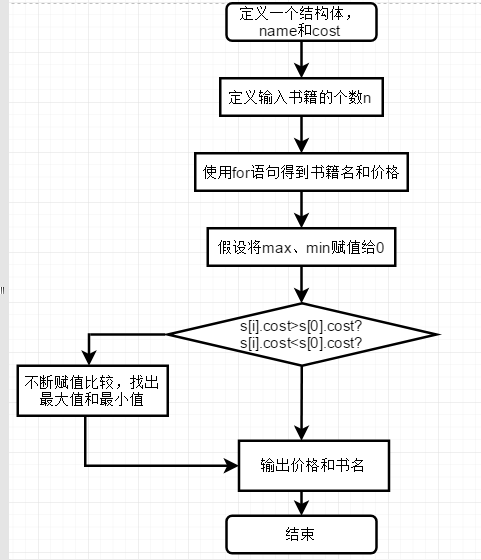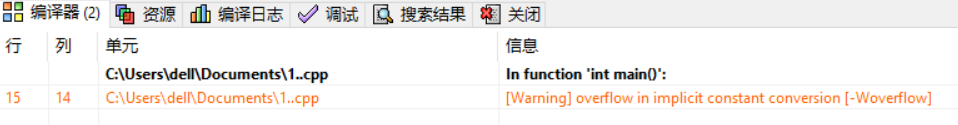# 第三次作业

1 设计思路
(1)主要描述题目算法

(2)流程图2.实验代码

#include<stdio.h>
struct student{
int num;
char name;
};
int main()
{
int N,i=0,num=0;
float aver=0;
scanf("%d",&N);
struct student s[N],*p;
p=s;
for(i=0;i<N;i++,p++)
{
}
if(N==0)
aver=0;
else
aver=num/(N*1.0);
printf("%.2f\n",aver);
p=s;
for(i=0;i<N;i++,p++)
{
printf("%s %.5d\n",p->name,p->num);
}
}

3.本题调试过程碰到问题及解决办法
错误原因：结构体大括号后面忘记分号。改正方法：和老师上课的代码进行比较。

1 设计思路
(1)主要描述题目算法

(2)流程图2.实验代码

#include <stdio.h>
#include <stdlib.h>
#include <string.h>
#include <math.h>

int main()
{
struct book
{
char name;
double price;
}now,max,min;

max.price=0;
min.price=999999999999999999;
int n,i;
scanf("%d",&n);
for(i=1;i<=n;i++)
{
getchar();
gets(now.name);
scanf("%lf",&now.price);
if(now.price>max.price)
{
strcpy(max.name,now.name);
max.price=now.price;
}
if(now.price<min.price)
{
strcpy(min.name,now.name);
min.price=now.price;
}
}
printf("%.2lf, %s\n%.2lf, %s",max.price,max.name,min.price,min.name);

return 0;
}

3.本题调试过程碰到问题及解决办法
错误原因：定义价格的时候使用了整型。
改正方法：警告我而且输出的东西乱七八糟然后仔细看了一下题目。1、总结两周来所有PTA作业中每个题目所使用的知识点。

2、将PTA作业的源代码使用git提交到托管平台上，要求给出上传成功截图和你的git地址。

https://git.coding.net/wangjiale0616/diyizhouzuoye.gitposted @ 2018-04-22 11:19  三水儿  阅读(181)  评论(0编辑  收藏  举报